# Pytorch 数据加载的分析

###### 来源：本文授权转自知乎作者巽二，https://zhuanlan.zhihu.com/p/100762487。未经作者许可，不得二次转载。

Pytorch数据加载的效率一直让人头痛，此前我介绍过两个方法，实际使用后数据加载的速度还是不够快，我陆续做了一些尝试，这里做个简单的总结和分析。

# 1、定位问题

• 读取图片数据（IO，可能存在IO瓶颈）
• 解码数据（一般是numpy格式，可能存在计算性能瓶颈）
• 数据增强（可能存在计算性能瓶颈）
• 类型变换（CPU->GPU，，可能存在数据拷贝瓶颈）

• 更快的图片解码
• 更快的数据增强
• 更强性能的设备，如使用GPU进行数据解码和增强（DALI库）

• CPU: Intel(R) Xeon(R) CPU E5-2698 v4 \@ 2.20GHz (正常负载，利用率小于30\%)
• GPU: V100 (正常负载，利用率小于30\%)
• 数据集数据840张，尺寸为1920x1080
• batch size = 64
• 无特别说明下num_workers=2
• 迭代10个epoch（13x10个迭代次数）

# 2. Baseline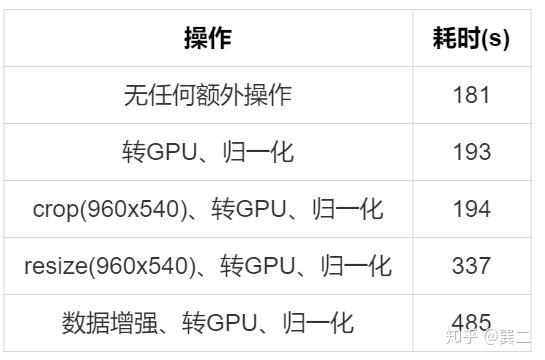• 无任何额外操作的输出图片为原始大小（1920x1080）
• 归一化的具体操作为： x = x.permute(0, 3, 1, 2).float().div(255)
• 转GPU的具体操作为： x = x.cuda()
• resize为opencv的resize，插值方式为 cv2.INTER_LINEAR
• 数据增强的操作使用Numpy和Opencv，包括：
• random resize
• random crop
• random filp
• random HSV

(1) num_workers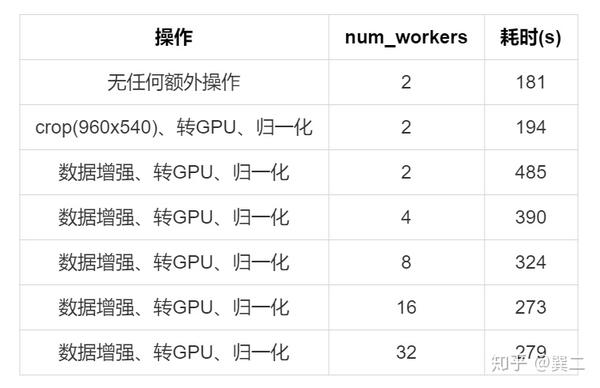(2) pin_memory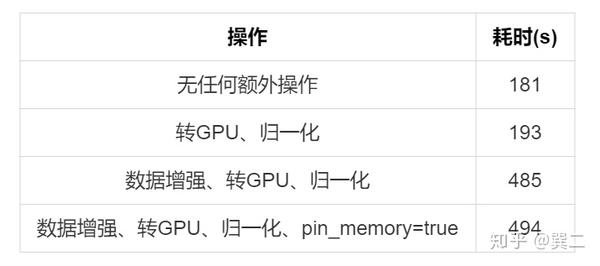(3) non_blocking

1. x = x.cuda(non_blocking=True)
2. 进行一些和x无关的操作
3. 执行和x有关的操作

non_blocking=true下，1不会阻塞212并行。但我们已经知道x.cuda()实际上耗时并不多（181s -> 194s，并不算是耗时的主要原因，就算去掉也不能加速太多。

class DataPrefetcher():
def __init__(self, dataset, batch_size=64, shuffle=True, num_workers=2):
dataset=dataset,
batch_size=batch_size,
shuffle=shuffle,
pin_memory=True,
num_workers=num_workers,
drop_last=True)
self.stream = torch.cuda.Stream()

try:
except StopIteration:
with torch.cuda.stream(self.stream):
self.next_x = self.next_x.cuda(non_blocking=True)
self.next_y = self.next_y.cuda(non_blocking=True)
self.next_x = self.next_x.permute(0, 3, 1, 2).float().div(255)
self.next_y = self.next_y.float()

def next(self):
torch.cuda.current_stream().wait_stream(self.stream)
data = (self.next_x, self.next_y)
return data

DataPrefetcher对DataLoader又包了一层，需要注意pin_memory=Truenon_blocking=true才才生效，next()函数直接返回data而无需等待cuda()。实验结果如下，和预期的差不多，并无明显的改善。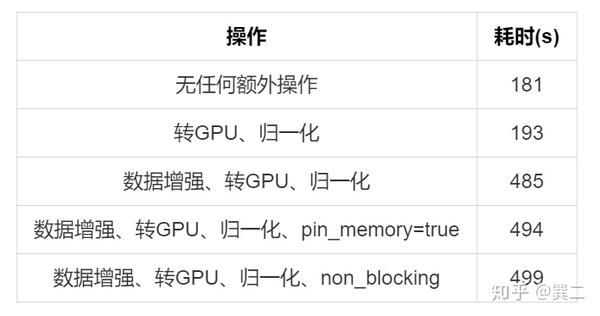1. x = x.cuda().permute(0, 3, 1, 2).float().div(255)
2. x = x.permute(0, 3, 1, 2).float().div(255).cuda()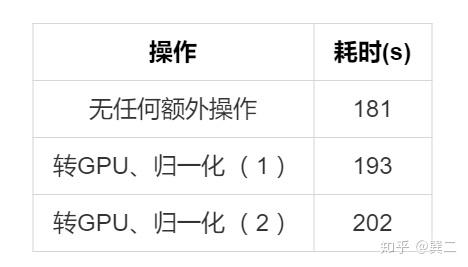# 4. 读取图片

(1) lmdb 先分析一下lmdb，一个jpg转lmdb的例子：

import numpy as np
import cv2
import os
import lmdb

list_path = "img_list/test.txt"
img_root = './data/test/edu/test/JPEGImages'
img_type = ".jpg"
label_root = './data/test/edu/test/labels'
label_type = ".txt"

env_db = lmdb.open("./test", map_size=1024*1024*1024*8)
txn = env_db.begin(write=True)

for line in open(list_path):
img_name = line.strip() + img_type
img_path = os.path.join(img_root, img_name)

label_name = line.strip() + label_type
label_path = os.path.join(label_root, label_name)

print(img_name)

# txn.put(img_name.encode(), img) ### 不编码
txn.put(img_name.encode(), cv2.imencode('.jpg', img))
txn.put(label_name.encode(), label)

txn.commit()
env_db.close()

img = np.frombuffer(self.txn.get(img_path.encode()), dtype=np.uint8)

label = np.frombuffer(self.txn.get(label_path.encode())).reshape(-1, 5) #lmdb存储后numpy会丢失形状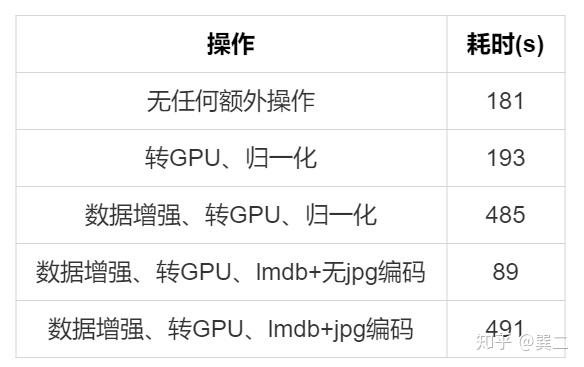(2) hdf5

f = h5py.File('test.h5', 'w')
img_dataset = f.create_dataset('img', shape=(840, 1080, 1920, 3), maxshape=(None, 1080, 1920, 3), chunks=(1, 1080, 1920, 3), dtype=np.uint8)
for i, line in enumerate(open(list_path)):
img_name = line.strip() + img_type
img_path = os.path.join(img_root, img_name)
img_dataset[i, :] = img
print(img_name, img.shape)
f.close()

f = h5py.File('test.h5', "r", swmr=True)
# f = h5py.File('test.h5', "r")
for i in range(840):
img = f["img"][i, ...](3) libjpeg-turbo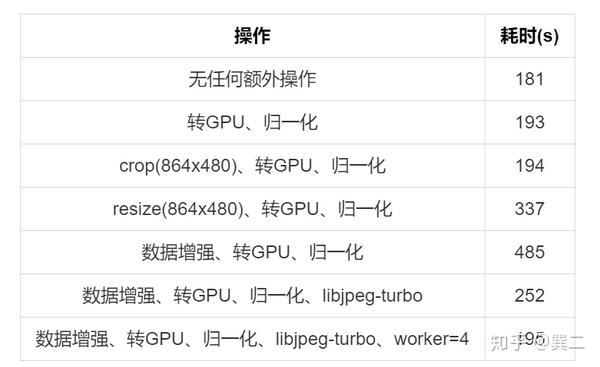(4) mxnet

mxnet的读取也测试了一下，测试时机器CPU占用发生变化，【数据增强、转GPU、归一化、libjpeg-turbo 】重新测试了一下，速度上无优势。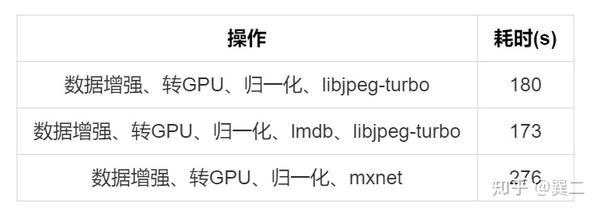(5) opencv缩小比例读取

img = cv2.imread(img_path, cv2.IMREAD_REDUCED_COLOR_2)
img = cv2.imread(img_path, cv2.IMREAD_REDUCED_COLOR_8)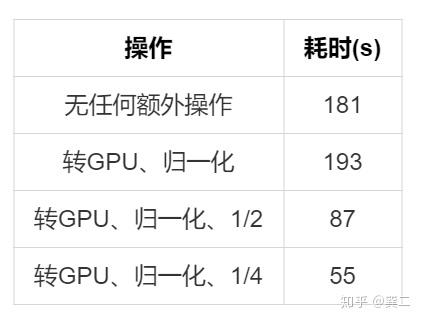# 5. 数据增强

(1) 建议 这里先给大家一些建议：

• opencv一般要比 PIL 快
• 由于数据增强操作基本是opencv的内置函数，因此cuda的\@jit加速是无效的，Cython应该也无加速效果。几个操作的耗时排列应该是这样的，opencv \<Cython \<jit \<pyhton。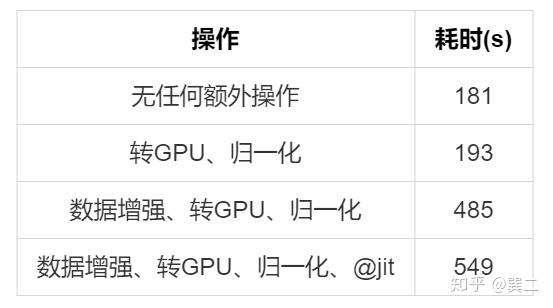• cv2.UMat()加速几乎无效，测试与不使用无差别，本地测试单图反而比不使用耗时长。
• 由于图片数据为0\~255，部分数值变换可以使用cv2.LUT()查找表直接映射,避免多次计算数值。
• 尽量提前做好数据处理，减少预处理步骤。

(2) DALI 如果加速还不满意的话，最后还有一个大招，DALLI库，大力出奇迹，极度推荐。 我们已经知道瓶颈在CPU的性能上，把这些计算放到GPU上是很合理的。NVIDIA DALI是一个GPU加速的数据增强和图像加载库，支持单个和批处理图像的解码、缩放、Crop、颜色空间转换等，具体支持的操作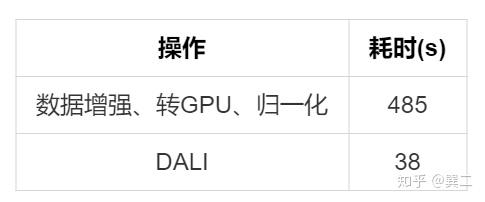pipeline = DataPipeline(**dataset_dict)
pipeline.build()
loader = DALIGenericIterator(pipeline, ["imgs", "labels"], img_len, fill_last_batch=False)

for j in range(epoch):
loader.reset()